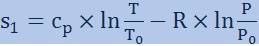# Entropy in isothermal expansion

Air:
V1= 0,1 m3
P1= 1 MPa
T1= 20 C
After isothermal expansion P2= 0,1 MPa

I had to find T2, M, V2, L, Q and found all those (T2=20 C; M=1,1927 kg; V2=1 m3; L=Q=230258 J) but need s (enthropy) for creating illustration in T-s
I cant find how its possible to calculate s1 and s2, is it possible at all or is it possible to find only delta s?